## 12.30.2012

### Connect MySql Database to Server

The connection of mysql database to server using object oriented concept, I uses these files:

1. connection.php
2. mysql.php
3. index.php
So, Now let's start:

First of all create a root_folder that will contains all of files says: eshop
Now open your favorite text-editor like as Adobe Dreamweaver and create a php file names connection.php and save it in our root folder as eshop.

connection.php

<?php
define'DB_SERVER' , 'localhost' );
define'DB_USER' , 'root' );
define'DB_PASSWORD' , '' );
define'DB_NAME' , 'eshop_db' );
?>

## 12.29.2012

### Number Rectangle

Q. Write a C program to print the following number rectangle:

1333
2222
3331

Ans.

/*c program for print number rectangle pyramid*/
#include<stdio.h>
int main()
{

## 12.17.2012

### Number Pyramid

Q. Write a C program to print the following number pyramid:

1
123
12345
1234567

Ans.

/*c program for number pyramid*/
#include<stdio.h>
int main()
{

## 12.09.2012

### Number Pryamid By Function

Q. Write a C program to print the following triangle using function.

1
2 3
4 5 6
7 8 9 1
2 3 4 5 6

Ans.

/*C program for number pyramid using function*/
#include<stdio.h>
void pyramid(int );
int main()
{

## 12.03.2012

### Character Rectangle Program

Q. Write a C program to print the following character pyramid as:

ABCD
BCDA
CDAB
DABC

Ans.

Cross-reference: Before you read answer of above program, i recommend to read Number Rectangle Program for easily understand this program.

/*c program for character pyramid*/
#include<stdio.h>
int main()

### Number Rectangle Program

Q. Write a C program to print the following number pyramid as:

1234
2341
3412
4123

Ans.

/*c program for number pyramid*/
#include<stdio.h>
int main()
{

### Character Pyramid Program

Q. Write a C program to print the following character pyramid C program:

ABCD

CDA
AB
C

Ans.

Cross-reference: Before you read answer of above program i recommend to read Number Pyramid Program for easily understand this program.

/*c program for character pyramid*/
#include<stdio.h>
int main()
{

### Number Pyramid

Q. Write a C program to print the following number pyramid C program:

1234
341
12
3

Ans.

/*c program for number pyramid*/
#include<stdio.h>
int main()
{

## 12.02.2012

### Character Rectangle Design

Q. Write a C program to print the following character rectangle structure:

ABCBA
AB BA
A   A
AB BA
ABCBA

Ans.

Cross-Reference: Before you reading answer of above program, i recommend to read Number Rectangle Design for easily understand this program.

/*c program for character rectangle pyramid*/
#include<stdio.h>
int main()
{

### Star Triangle Pyramid

Q. Write a C program to print the following star pyramid structure:

*********
*******
*****
***
*

Ans.

/*c program for star pyramid design*/
#include<stdio.h>
int main()
{

### Number Rectangle Design

Q. Write a C program to print the following number rectangle design :

12321
12 21
1   1
12 21
12321

Ans.

/*c program for number rectangle design*/
#include<stdio.h>
int main()
{

### Number Triangle Program

Q. Write a C program to print the following number triangle design:

1
232
34543
4567654

Ans.

/*c program for number triangle design*/
#include<stdio.h>
int main()
{

## 11.19.2012

### Star Pyramid

Q. Write a C program to accept the number of rows of pyramid from user and print the correspondence star triangle.
For example,

Enter number by user : 7

*
**
***
****
***
**
*

Ans.

/*c program for star pyramid*/
#include<stdio.h>
int main()
{
int num,r,c;
printf("Enter number of rows : ");
scanf("%d", &num);
for(r=1; r<=(num/2)+1; r++)
{
for(c=1; c<=r; c++)
printf("*");
printf("\n");
}
for(r=1; r<=(num/2); r++)
{
for(c=r; c<=num/2; c++)
printf("*");
printf("\n");
}
return 0;
}

The output of above program would be:Figure: Screen shot for star pyramid C program

## 11.18.2012

### Character Pyramid

Q. Write a C program to print the following character pyramid:

A
BA
ABA
BABA
ABABA

Ans.

/*c program for character pyramid*/
#include<stdio.h>
int main()
{
int num,r,c;
char ch='A',st='B';
printf("Enter number of rows: ");
scanf("%d", &num);
for(r=1; r<=num; r++)
{
for(c=r; c>=1; c--)
{
if(c%2==0)
printf("%c", st);
else
printf("%c", ch);
}
printf("\n");
}
return 0;
}

The output of above program would be: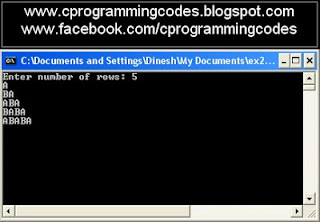Figure: Screen shot for character pyramid C program

## 11.10.2012

### User Define Function- Power

Q. Write a C program to create function power and calculate the power of any number.
For example:
Assume number = 5
Power = 3
Result = 5*5*5 = 125

Ans.

/*c program for creating user define function power and calculating power of number*/
#include<stdio.h>
int power(int , int );
int main()
{
int num,pow,res;   //res = result
printf("Enter any number : ");
scanf("%d", &num);
printf("Enter power of number : ");
scanf("%d", &pow);
res = power(num,pow);
printf("%d's power %d = %d",num,pow,res);
return 0;
}

int power(int n, int p)
{
int r=1;
for(; p>=1; p--)
r = r*n;
return r;
}

The output of above program would be:Figure: Screen shot for calculating power of any number using user define function power C program

### User Define Function- Reverse Number

Q. Write a C program to create a function that would reverse the any number.
For example:
Assume enter number = 57429
Result = 92475

Ans.

/*c program for reverse number using user define function- rev */
#include<stdio.h>
int rev(int );
int main()
{
int num,res;  // res = result
printf("Enter any number : ");
scanf("%d", &num);
res = rev(num);
printf("Reverse order number = %d",res);
return 0;
}

int rev(int n)
{
int r=0;
for(; n>=1; n=n/10)
r = r*10 + n%10;
return r;
}

The output of above program would be:Figure: Screen shot for reverse digit using user define function C program

### User Define Function- LeapYear

Q. Write a C program to find entered year is leap year or not using own created function say "leap".

Ans.

/*c program for find year is leap year or not using user define function leap*/
#include<stdio.h>
int leap(int );
int main()
{

## 11.05.2012

### User Define Function- Factorial

Q. Write a function fact to calculate the factorial value of any number.

Ans.

/*c program for make function fact to calculate factorial value of any number*/
#include<stdio.h>
int fact(int );
int main()
{

## 11.04.2012

### Number Pyramid

Q. Write a C program to print the following number pyramid :

1 2 3 4 5
1 2 3 4
1 2 3
1 2
1

Ans.

/*c program for number pyramid*/
#include<stdio.h>
int main()
{
int num,n,r,c,sp;
printf("Enter any number : ");
scanf("%d", &num);
n=num;
for(r=1; r<=num; r++,n--)
{
for(sp=1; sp<r; sp++)
printf(" ");
for(c=1; c<=n; c++)
printf("%d ",c);
printf("\n");
}
return 0;
}

The output of above program would be:Figure: Screenshot for number pyramid C program

### Number Pyramid

Q. Write a C program to print the following number pyramid as:

1 2 3 4 5
2 3 4 5
3 4 5
4 5
5

Ans.

/*c program for number pyramid*/
#include<stdio.h>
int main()
{
int num,r,c,sp;
printf("Enter any number : ");
scanf("%d", &num);
for(r=1; r<=num; r++)
{
for(sp=1; sp<r; sp++)
printf(" ");
for(c=r; c<=num; c++)
printf("%d ",c);
printf("\n");
}
return 0;
}

The output of above program would be: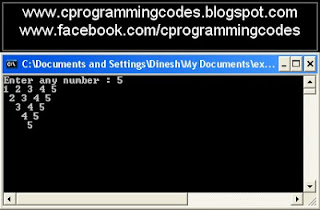Figure: Screenshot for number pyramid C program

## 11.03.2012

### Floyd Triangle

Q. Write a C program to print the following number pyramid or Floyd triangle:

1
2 3
4 5 6
7 8 9 10

Ans.

/*c program to print the number pyramid*/
#include<stdio.h>
int main()
{
int num,r,c,sp,i=1;
printf("Enter any number : ");
scanf("%d", &num);
for(r=1; r<=num; r++)
{
for(sp=1; sp<=num-r; sp++)
printf(" ");
for(c=1; c<=r; c++,i++)
printf("%d ",i);
printf("\n");
}
return 0;
}

The output of above program would be:Figure: Screen shot for Floyd triangle C program

## 11.02.2012

### Flowchart for Fibonacci Series

Q. Draw the flowchart of Fibonacci series C program.

Ans.

Flowchart for Fibonacci series C program as: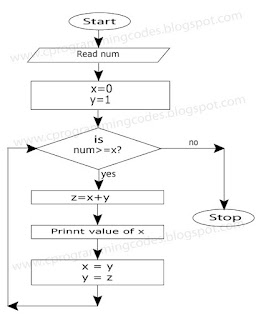Figure: Flowchart for generate Fibonacci series

Related programs:

### Number Character Pyramid

Q. Write a C program to print the following number character pyramid.

1
AB
123
ABCD
12345

Ans.

/*c program for number character pyramid*/
#include<stdio.h>
int main()
{
int num=5,r,c;
char ch;
for(r=1; r<=num; r++)
{
if(r==2 || r==4)
{
ch='A';
for(c=1; c<=r; c++,ch++)
printf("%c",ch);
}
else
{
for(c=1; c<=r; c++)
printf("%d",c);
}
printf("\n");
}
return 0;
}

The output of above program would be:Figure: Screen shot for number character pyramid C program

## 10.29.2012

### Number Pyramid

Q. Write a C program to print the following number pyramid:

54321
5432
543
54
5

Ans.

/*c program for number pyramid*/
#include<stdio.h>
int main()
{
int num,r,c;
printf("Enter any number: ");
scanf("%d", &num);
for(r=1; r<=num; r++)
{
for(c=num; c>=r; c--)
printf("%d",c);
printf("\n");
}
return 0;
}

The output of above program would be:Figure: Screen shot for number pyramid C program

## 10.22.2012

### Call by reference swapping flowchart

Q. Write a C program to give a example of swapping two values using call by reference method.
Also draw appropriate flowchart.

Ans.

C program for  call by reference swap method, click below link:
click me for call by reference swap C program

Call by reference swapping flowchart as:Figure: Flowchart for call by reference swap two values program

### Odd Number Series Pyramid

Q. Write a C program to print the odd number series pyramid.
or
Q. Write a C program to print the following number pyramid as:

1
3  5  7
9 11  13  15  17  19

Ans.

/*c program for odd number pyramid*/
#include<stdio.h>
int main()
{
int n=2,r,c,z=3;
printf("1\n");
for(r=1; r<=2; r++)
{
for(c=1; c<=r*3; c++,z=z+2)
printf("%d ",z);
printf("\n");
}
return 0;
}

The output of above program would be:Figure: Screen shot for odd number series pyramid C program

## 10.21.2012

### Reverse Star Pyramid

Q. Write a C program to print the following star structure:

**********
****  ****
***    ***
**      **
*        *

Ans.

/*C program for reverse star pyramid*/
#include<stdio.h>
#include<conio.h>
int main()
{
int num,c,sp,r=1;
printf("Enter loop repeat number(rows): ");
scanf("%d", &num);
printf("\n");
for(; num>=1; num--,r++)
{
for(c=1; c<=num; c++)
printf("*");
for(sp=r; sp>1; sp--)
printf(" ");
for(sp=r; sp>1; sp--)
printf(" ");
for(c=num; c>=1; c--)
printf("*");
printf("\n");
}
return 0;
}

The output of above program would be:Figure: Screen shot for reverse star pyramid C program

## 10.20.2012

### Odd Loop

What is odd loop?
In real life programming, there are many times comes a situation when we don't know how many times the statements in the loop are to be executed.
There is comes concept of odd loop.
Execution of loop an unknown number of times can be done by - while, for and do...while loops.

/*A demonstration of odd loop using do...while*/
#include<stdio.h>
int main()
{
int n;
char answer;
do
{
printf("Enter any number : ");
scanf("%d", &n);
printf("Square of %d is %d",n,n*n);
fflush(stdin);
printf("\nWant to calculate more square y/n: ");
scanf("%c", &answer);
}while(answer=='y');
return 0;
}

The output of above program would be: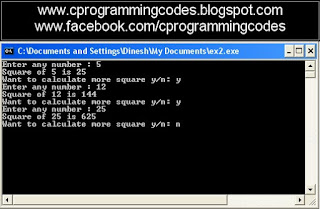Figure: Screen shot of odd loop (do...while) to calculate square of number C program

The above odd loop program we can write using for loop as:

/*odd loop using for loop of calculate square number C program*/

#include<stdio.h>
int main()
{
int n;
char ans='y';
for(; ans=='y' ; )
{
printf("Enter any number : ");
scanf("%d"&n);
printf("Square of %d is %d",n,n*n);
fflush(stdin);
printf("\nWant to calculate more square y/n: ");
scanf("%c"&ans);
}
return 0;
}

The output of above program would be:Figure: Screen shot of odd loop (for) to calculate square of number C program

The odd loop program we can write using while loop as:
/*odd loop using while loop of calculate square number C program*/

#include<stdio.h>
int main()
{
int n;
char ans='y';
while(ans=='y')
{
printf("Enter any number : ");
scanf("%d"&n);
printf("Square of %d is %d",n,n*n);
fflush(stdin);
printf("\nWant to calculate more square y/n: ");
scanf("%c"&ans);
}
return 0;
}

The output of above program would be: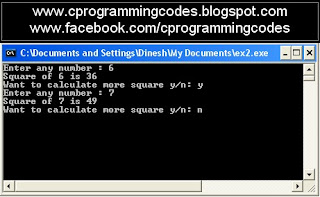Figure: Screen shot of odd loop ( while ) to calculate square of number C program